66 Shares

In this Python data analysis tutorial, we will focus on how to carry out between-subjects ANOVA in Python. As mentioned in an earlier post (Repeated measures ANOVA with Python) ANOVAs are commonly used in Psychology. We start with some brief introduction to the theory of ANOVA.

If you are more interested in the four methods to carry out one-way ANOVA with Python click here.

In this post we will learn how to carry out ANOVA using SciPy, calculating it “by hand” in Python, using Statsmodels, and Pyvttbl.

Update: the Python package Pyvttbl is not maintained for a couple of years but there’s a new package called Pingouin. As a bonus, how to use this package is added at the end of the post.

## Prerequisites

In this post, you will need to install the following Python packages:

• SciPy
• NumPy
• Pandas
• Statsmodels
• Pingouin

Of course, you don’t have to install all of these packages to perform the ANOVA with Python. Now, if you only want to do the data analysis you can choose to install either SciPy, Statsmodels, or Pingouin. However, Pandas will be used to read the example datasets and carry out some simple descriptive stats as well as visualization of the data. Installing Python packages can be done with either pip or conda, for example. Here’s how to install all of the above packages:

.wp-block-code {
border: 0;
}

.wp-block-code > div {
overflow: auto;
}

.shcb-language {
border: 0;
clip: rect(1px, 1px, 1px, 1px);
-webkit-clip-path: inset(50%);
clip-path: inset(50%);
height: 1px;
margin: -1px;
overflow: hidden;
position: absolute;
width: 1px;
word-wrap: normal;
word-break: normal;
}

.hljs {
box-sizing: border-box;
}

.hljs.shcb-code-table {
display: table;
width: 100%;
}

.hljs.shcb-code-table > .shcb-loc {
color: inherit;
display: table-row;
width: 100%;
}

.hljs.shcb-code-table .shcb-loc > span {
display: table-cell;
}

.wp-block-code code.hljs:not(.shcb-wrap-lines) {
white-space: pre;
}

.wp-block-code code.hljs.shcb-wrap-lines {
white-space: pre-wrap;
}

.hljs.shcb-line-numbers {
border-spacing: 0;
counter-reset: line;
}

.hljs.shcb-line-numbers > .shcb-loc {
counter-increment: line;
}

.hljs.shcb-line-numbers .shcb-loc > span {
}

.hljs.shcb-line-numbers .shcb-loc::before {
border-right: 1px solid #ddd;
content: counter(line);
display: table-cell;
text-align: right;
-webkit-user-select: none;
-moz-user-select: none;
-ms-user-select: none;
user-select: none;
white-space: nowrap;
width: 1%;
}
pip install scipy numpy pandas statsmodels pingouinCode language: Bash (bash)

Now, pip can also be used to install a specific version of a package. To install an older version you add “==” followed by the version you want to be installed. In the next section, you will get a brief introduction to ANOVA, in general. Of course, if you only plan to use one of the packages, you can install one of them. Note, however, if you install statsmodels with e.g. pip you will install also SciPy, NumPY, and Pandas.

## Introduction to ANOVA

Before we learn how to do ANOVA in Python, we are briefly discussing what ANOVA is. ANOVA is a means of comparing the ratio of systematic variance to unsystematic variance in an experimental study. Variance in the ANOVA is partitioned into total variance, variance due to groups, and variance due to individual differences.

The ratio obtained when doing this comparison is known as the F-ratio. A one-way ANOVA can be seen as a regression model with a single categorical predictor. This predictor usually has two plus categories. A one-way ANOVA has a single factor with J levels. Each level corresponds to the groups in the independent measures design.

The general form of the model, which is a regression model for a categorical factor with J levels, is:

$latex y_i = b_0+b_1X_{1,i} +…+b_{j-1,i} + e_i&s=2$

There is a more elegant way to parametrize the model. In this way, the group means are represented as deviations from the grand mean by grouping their coefficients under a single term.  I will not go into detail on this equation:

$latex y_{ij} = \mu_{grand} + \tau_j + \varepsilon_{ij}&s=2$

As for all parametric tests the data need to be normally distributed (each group’s data should be roughly normally distributed) for the F-statistic to be reliable. Each experimental condition should have roughly the same variance (i.e., homogeneity of variance), the observations (e.g., each group) should be independent, and the dependent variable should be measured on, at least,  an interval scale.

### Assumptions

As with all parametric tests also ANOVA has a number of assumptions. First of all, the groups have to be independent of each other. Second, the data needs to be normally distributed (within each group). Third, there have to be equal variances between all groups. Homogeneity of variances can be tested with Bartlett’s and Levene’s test in Python (e.g., using SciPy) and the normality assumption can be tested using the Shapiro-Wilks test or by examining the distribution. Note, if your data is skewed you can transform it using e.g. the log transformation in Python.

### A Priori Tests

When conducting ANOVA in Python, it is usually best to restrict the testing to a small set of possible hypotheses. Furthermore, these tests should be motivated by theory and are known as a priori or planned comparisons. As the names imply, these tests should be planned before the data is collected.

### Post-Hoc Tests (Pairwise Comparisons) in Python

Even though studies can have a strong theoretical motivation, as well as a priori hypotheses, there will be times when the pattern occurs after the data is collected. Note, if there are many possible tests, these post-hoc tests, the error rate is determined by the number of tests that might have been carried out.

There are a number of possible post-hoc tests that can be carried out. In this ANOVA in Python tutorial, we will use the Tukey’s honestly significant difference (Tukey-HSD) test

## 6 Steps to Carry Out ANOVA in Python

Now, before getting into details here are 6 steps to carry out ANOVA in Python:

1. Install the Python package Statsmodels (pip install statsmodels)
2. Import statsmodels api and ols:import statsmodels.api as sm  and from statsmodels.formula.api import ols
3. Import data using Pandas
4. Set up your model mod = ols('weight ~ group', data=data).fit()
5. Carry out the ANOVA: aov_table = sm.stats.anova_lm(mod, typ=2)
6. Print the results: print(aov_table)

Now, sometimes when we install packages with Pip we may notice that we don’t have the latest version installed. If we want to, we can of course, update pip to the latest version using pip or conda.

## ANOVA using Python

In the four Python ANOVA examples in this tutorial we are going to use the dataset “PlantGrowth” that originally was available in R. However, it can be downloaded using this link: PlantGrowth. In the first three examples, we are going to use Pandas DataFrame. All three Python ANOVA examples below are using Pandas to load data from a CSV file. Note, we can also use Pandas read excel if we have our data in an Excel file (e.g., .xlsx).

import pandas as pd

datafile = "PlantGrowth.csv"

#Create a boxplot
data.boxplot('weight', by='group', figsize=(12, 8))

ctrl = data['weight'][data.group == 'ctrl']

grps = pd.unique(data.group.values)
d_data = {grp:data['weight'][data.group == grp] for grp in grps}

k = len(pd.unique(data.group))  # number of conditions
N = len(data.values)  # conditions times participants
n = data.groupby('group').size() #Participants in each conditionCode language: Python (python)

Judging by the Boxplot there are differences in the dried weight for the two treatments. However, easy to visually determine whether the treatments are different from the control group.

### ANOVA in Python using SciPy

We start this Python ANOVA tutorial using SciPy and its method f_oneway from stats.

from scipy import stats

F, p = stats.f_oneway(d_data['ctrl'], d_data['trt1'], d_data['trt2'])Code language: Python (python)

One problem with using SciPy is that following APA guidelines we should also effect size (e.g., eta squared) as well as Degree of freedom (DF). DFs needed for the example data is easily obtained

DFbetween = k - 1
DFwithin = N - k
DFtotal = N - 1Code language: Python (python)

However, if we want to calculate eta-squared we need to do some more computations. Thus, the next section will deal with how to calculate a one-way ANOVA using the Pandas DataFrame and Python code.

### Calculating using Python (i.e., pure Python ANOVA)

A one-way ANOVA in Python is quite easy to calculate so below I am going to show how to do it. First, we need to calculate the sum of squares between (SSbetween), sum of squares within (SSwithin), and sum of squares total (SSTotal).

#### Sum of Squares Between

We start by calculating the Sum of Squares between. Sum of Squares Between is the variability due to interaction between the groups. Sometimes known as the Sum of Squares of the Model.

$latex SSbetween = \frac{\sum(\sum k_i) ^2} {n} – \frac{T^2}{N}&s=2$

SSbetween = (sum(data.groupby('group').sum()['weight']**2)/n) \
- (data['weight'].sum()**2)/NCode language: Python (python)

#### How to Calculate the Sum of Squares Within

The variability in the data due to differences within people. The calculation of Sum of Squares Within can be carried out according to this formula:

$latex SSwithin = \sum Y^2 – \frac{\sum (\sum a_i)^2}{n}&s=2$

sum_y_squared = sum([value**2 for value in data['weight'].values])
SSwithin = sum_y_squared - sum(data.groupby('group').sum()['weight']**2)/nCode language: Python (python)

#### Calculation of Sum of Squares Total

Sum of Squares Total will be needed to calculate eta-squared later. This is the total variability in the data.

$latex SStotal = \sum Y^2 – \frac{T^2}{N}&s=2$

SStotal = sum_y_squared - (data['weight'].sum()**2)/NCode language: Python (python)

#### How to Calculate Mean Square Between

Mean square between is the sum of squares within divided by degree of freedom between.

MSbetween = SSbetween/DFbetween

#### Calculation of Mean Square Within

Mean Square within is also an easy calculation;

MSwithin = SSwithin/DFwithinCode language: Python (python)

#### Calculating the F-value

F = MSbetween/MSwithinCode language: Python (python)

To reject the null hypothesis we check if the obtained F-value is above the critical value for rejecting the null hypothesis. We could look it up in an F-value table based on the DFwithin and DFbetween. However, there is a method in SciPy for obtaining a p-value.

p = stats.f.sf(F, DFbetween, DFwithin)Code language: Python (python)

Finally, we are also going to calculate the effect size. We start with the commonly used eta-squared (η² ):

eta_sqrd = SSbetween/SStotalCode language: Python (python)

However, eta-squared is somewhat biased because it is based purely on sums of squares from the sample. No adjustment is made for the fact that what we aiming to do is to estimate the effect size in the population. Thus, we can use the less biased effect size measure Omega squared:

om_sqrd = (SSbetween - (DFbetween * MSwithin))/(SStotal + MSwithin)Code language: Python (python)

The results we get from both the SciPy and the above method can be reported according to APA style; F(2, 27) = 4.846, p =  .016, η² =  .264. If you want to report Omega Squared: ω2 = .204. That was it, now we know how to do ANOVA in Python by calculating everything “by hand”.

### ANOVA in Python using Statsmodels

In this section of the Python ANOVA tutorial, we will use Statsmodels. First, we start by using the ordinary least squares (ols) method and then the anova_lm method. Also, if you are familiar with R-syntax, Statsmodels have a formula APIwhere our model is very intuitively formulated.

Here’s three simple step for carrying out ANOVA using Statsmodels:

Time needed: 1 minute.

In the ANOVA how-to below, it is assumed that the data is in a Pandas dataframe (i.e., df).

1. Import the needed Python packages

First, we import statsmodels API and ols: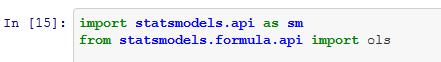2. Set up the ANOVA model

Second, we use ols to set up our model using a formula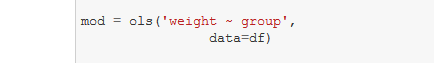3. Carry out the ANOVA

We can now use anova_lm to carry out the ANOVA in Python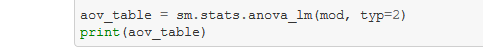In the ANOVA example below, we import the API and the formula API. Second, we use ordinary least squares regression with our data. The object obtained is a fitted model that we later use with the anova_lm method to obtain an ANOVA table.

In the final part of this section, we are going to carry out pairwise comparisons using Statsmodels.

import statsmodels.api as sm
from statsmodels.formula.api import ols

mod = ols('weight ~ group',
data=data).fit()

aov_table = sm.stats.anova_lm(mod, typ=2)
print(aov_table)Code language: Python (python)

#### ANOVA Python table:

Note, no effect sizes are calculated when we use Statsmodels.  To calculate eta squared we can use the sum of squares from the table:

esq_sm = aov_table['sum_sq']/(aov_table['sum_sq']+aov_table['sum_sq'])aov_table['EtaSq'] = [esq_sm, 'NaN']print(aov_table)Code language: Python (python)

###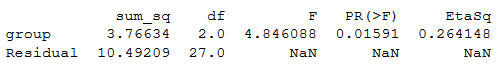Save

#### Python ANOVA: Pairwise Comparisons

It is, of course, also possible to calculate pairwise comparisons for our Python ANOVA using Statsmodels. In the next example, we are going to use the t_test_pairwise method. Conducting post-hoc tests, corrections for familywise error can be carried out using a number of methods (e.g., Bonferroni, Šidák)

pair_t = mod.t_test_pairwise('group')
pair_t.result_frameCode language: Python (python)

Note, if we want to use another correction method, we add the parameter method and add “bonferroni” or “sidak”, for instance (e.g., method=”sidak”).

If we were to carry out regression analysis, using Python, we might have to convert the categorical variables to dummy variables using Pandas get_dummies() method.

### Python ANOVA using pyvttbl anova1way

In this section, we are going to learn how to carry out an ANOVA in Python using the method anova1way from the Python package pyvttbl. This package also has a DataFrame method. We have to use this method instead of Pandas DataFrame to be able to carry out the one-way ANOVA in Python.  Note, Pyvttbl is old and outdated. It requires Numpy to be at most version 1.1.x or else you will run into an error ( “unsupported operand type(s) for +: ‘float’ and ‘NoneType’”).

This can, of course, be solved by downgrading Numpy (see my solution using a  virtual environment Step-by-step guide for solving the Pyvttbl Float and NoneType error). However, it may be better to use pingouin for carrying out Python ANOVAs (see the next section of this blog post).

from pyvttbl import DataFrame

df=DataFrame()
aov_pyvttbl = df.anova1way('weight', 'group')
print aov_pyvttblCode language: Python (python)

#### Output anova1way

Anova: Single Factor on weight

SUMMARY
Groups   Count    Sum     Average   Variance
============================================
ctrl        10   50.320     5.032      0.340
trt1        10   46.610     4.661      0.630
trt2        10   55.260     5.526      0.196

O'BRIEN TEST FOR HOMOGENEITY OF VARIANCE
Source of Variation    SS     df    MS       F     P-value   eta^2   Obs. power
===============================================================================
Treatments            0.977    2   0.489   1.593     0.222   0.106        0.306
Error                 8.281   27   0.307
===============================================================================
Total                 9.259   29

ANOVA
Source of Variation     SS     df    MS       F     P-value   eta^2   Obs. power
================================================================================
Treatments             3.766    2   1.883   4.846     0.016   0.264        0.661
Error                 10.492   27   0.389
================================================================================
Total                 14.258   29

POSTHOC MULTIPLE COMPARISONS

Tukey HSD: Table of q-statistics
ctrl     trt1       trt2
=================================
ctrl   0      1.882 ns   2.506 ns
trt1          0          4.388 *
trt2                     0
=================================
+ p < .10 (q-critical[3, 27] = 3.0301664694)
* p < .05 (q-critical[3, 27] = 3.50576984879)
** p < .01 (q-critical[3, 27] = 4.49413305084)Code language: HTTP (http)

We get a lot of more information using the anova1way method. What may be of particular interest here is that we get results from a post-hoc test (i.e., Tukey HSD).  Whereas the ANOVA only lets us know that there was a significant effect of treatment the post-hoc analysis reveals where this effect may be (between which groups).

If you have more than one dependent variable a multivariate method may be more suitable. Learn more on how to carry out a Multivariate Analysis of Variance (ANOVA) using Python:

### Python ANOVA using Pingouin (bonus)

In this section, we are going to learn how to carry out ANOVA in Python using the package pingouin. This package is, as with Statsmodels, very simple to use. If we want to carry out an ANOVA we just use the method called anova.

import pandas as pd
import pingouin as pg

data = "https://vincentarelbundock.github.io/Rdatasets/csv/datasets/PlantGrowth.csv"

aov = pg.anova(data=df, dv='weight', between='group', detailed=True)
print(aov)Code language: Python (python)

As can be seen in the ANOVA table above, we get the degrees of freedom, the mean square error, F- and p-values, as well as the partial eta squared when using pingouin.

#### Pairwise Comparisons  in Python (Tukey-HSD)

One neat thing with Pingouin is that we can also carry post-hoc tests. We are now going to carry out the Tukey-HSD test as a follow up on our ANOVA. This is also very simple we use the pairwise_tukey method to carry out the pairwise comparisons:

pt = pg.pairwise_tukey(dv='weight', between='group', data=df)
print(pt)Code language: Python (python)

##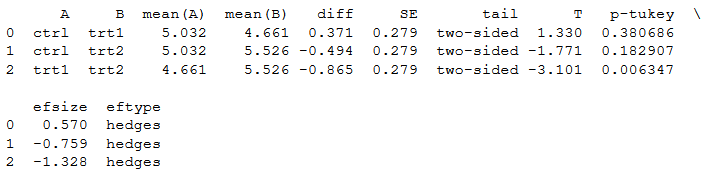Save

Note, if we want another type of effect size we can add the argument effsize and choose between six different effect sizes (or none): cohen, hedges, glass, eta-square, odds-ratio, and AUC. In the last code example we change the default effect size (hedges) to cohen:

cpt = pg.pairwise_tukey(dv='weight', between='group', effsize='cohen', data=df)
print(pt)Code language: Python (python)

## Conclusion: Python ANOVA

That is it! In this tutorial you learned 4 methods that let you carry out one-way ANOVAs using Python. There are, of course, other ways to deal with the tests between the groups (e.g., the post-hoc analysis).

One could carry out Multiple Comparisons (e.g., t-tests between each group. Just remember to correct for familywise error!) or Planned Contrasts.  In conclusion, doing ANOVAs in Python is pretty simple.66 Shares